• 검색 결과가 없습니다.

# Reservoir computing based on explosive synchronization and quenched chaos

N/A
N/A
Protected

Share "Reservoir computing based on explosive synchronization and quenched chaos"

Copied!
32
0
0
더 보기 ( 페이지)

전체 글

In this work, we present a reservoir computer (RC), a highly efficient bio-inspired architecture, based on oscillator synchronization in a critical regime. One of the advantages of adopting coupled phase oscillators as neuromorphic elements is that the synchrony between oscillators can be fine-tuned at artificial condition. The proposed framework shows better results in signal reconstruction tasks than RC based on explosive synchronization of regular phase oscillators.

We have also shown that the information capacity of reservoirs at the critical point can be used as a predictive measure for the computational capacity of the reservoir. Recently, reservoir computing has emerged as a promising computational framework for applying dynamic system computing. The main advantage of reservoir computing is their simplicity in the training process compared to other neural networks.

In the case of a reservoir based on continuous dynamical systems, one can create criticality by setting intrinsic parameters so that the reservoir operates at a bifurcation point beyond which the dimension of the attractor suddenly decreases. In the second section, we show that a reservoir of coupled Kuramoto oscillators performs excellent computations in a critical regime near explosive synchronization. The proposed reservoir uses transient dynamics of a coupled chaotic oscillators in a critical regime where sudden amplitude death occurs.

And we checked that it can be a reliable measure for reservoir calculation based on dynamic oscillators.

## Model : Kuramoto Oscillators

The explosive synchronization occurs with hysteresis: besides the forward transition from the incoherent state to the phase-locked states, there is also an abrupt desynchronization with decrease of the coupling strength, which does not overlap with the forward transition. However, we only focus on the forward bifurcation in neuromorphic computing, as we need to keep the system out of the hysteresis loop, to avoid the risk of permanent desynchrony. The plot (red circles) in Figure 1(a) shows that forward discontinuous phase transition occurs at a critical coupling strength λ ≈ 2.9, which makes a striking difference from the continuous phase transition at λ≈1.6.

In this work we compare the two computational reservoirs based on the Kuramoto model (1) that use the different settings for the coupling strength and the network topology: .. 2) Explosive synchronization model(ES) coupling strength: λi =λ|ωi | λ >0,. In Kuramoto-based models, higher coupling strength causes the oscillators to be synchronized in a tighter phase-locked state. For balanced calculations, it is reasonable to set the coupling strength at which the phase of the oscillators are marginally locked and can respond quickly to external stimuli.

The Kuramoto order parameter r can be used as an indicator for the critical value of the coupling strength λ. For reliable calculations, the time variance of the frequency must be low in the ground state. Note that for each oscillator, the time-frequency variance becomes 0 when the oscillators are in a phase-locked state, keeping their common frequency constant.

In the case of ES, the acceptable range for c is 102 ≤c ≤ 108„, regardless of the network topology of coupled oscillators. Although rvar does not explicitly show the difference between explosive and non-explosive synchronizations, it provides clear information about a level of coupling strength for which oscillators are designed for reliable calculations. From here we will use the variance order parameter rvar to examine the relationship between the states of the system and its computing performance.

All the evolutionary activities of the oscillators are collected through the frequency values ​​θi0(t) and assigned to the desired output by an output function error = (err1 , · · ·, errorq )∈Rq. In the reading process, it is better to use not only the past values ​​of the nodes, but also the current values, to exploit the rich dynamics of the chaotic reservoirs. Here we use an output function that takes s sampled states of the system at discrete times t−∆t, t− 2∆t, · · · , t−s∆ and maps them to the desired output at time.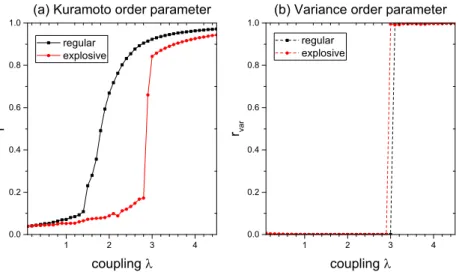Figure 1: Kuramoto and variance order parameters according to the coupling strength: RS and ES use the complete network and a Erdős-Rényi graph with the mean degree hk i i = 6, respectively

## Numerical Tests

Having observed the optimized computational capability of RS at criticality, we now turn to the case where the critical transition occurs with the explosive synchronization. However, one can see that the accuracy has greatly improved by 10 to 1000 times, compared to that of RS. Another observation is that the error level maintains even for stronger coupling forces beyond the critical point, while it slowly increases in RS in Figure 2.

It is assumed that the computer's ability to handle various input signals in different tasks is closely related to the spectral properties of the system responding to disturbances. This implies that the system is well prepared for different tasks involving a wide range of wavelengths. In this section, we investigate ES in the random networks with various levels of connectivity.

It can be seen that the dual-task performance is minimized when the average scale is about 6 to 24. On the contrary, it is observed in Figure 6 that the densely coupled oscillators do not perform well either. A practical alternative is to measure the training error from the scores instead of estimating the order parameters.

That is, one can detect a critical coupling strength from a sudden change in the training error. Note that the training error is kept as high as the test error until the coupling strength reaches a certain level, which means that RS hardly learns from the training set in a weak coupling regime. Interestingly, in Figure 8, the more dramatic and precisely opposite situation occurs with ES: the training error stays low and makes a sharp increase at the critical point.

A coupling strength less than a critical value appears to cause the tank to simply reproduce the training data but fail to process the new data. The reservoir achieves the ability to find the right task pattern only when the coupling power is in the first-order criticality regime. In contrast to RS, the sudden increase in training error in ES indicates that the coupling strength reaches a critical level for synchronization.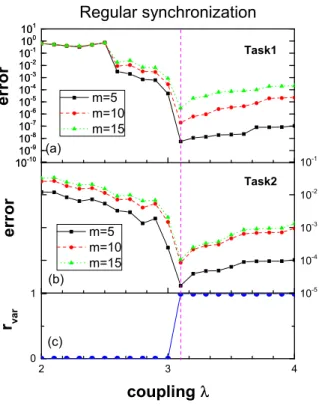Figure 2: Test error according to the coupling strength in RS. The sudden changes in error in (a) and (b) coincides with the criticality in the variance order parameter in (c).

## Model : Coupled Lorenz Oscillators

Adjusting the parameters according to the order parameter will be discussed in Section 3. The advantage of using the coupled chaotic systems in (9) as a reservoir is that we can easily create a large criticality with a first-order phase transition in the system. Beyond the critical point of the coupling force, the compound oscillatory motions of the system collapse into an equilibrium point.

This fixed equilibrium state near a critical point is used as the ground state for reservoir calculations, to which the system always returns after each calculation, clearing unnecessary information from previous evaluations and preparing for the next input. To look for a possible phase transition in (9), we take the order parameter rvar in terms of the variation of amplitudes, as defined in the previous section (3). To be more certain, we propose the commonly used order parameter for chaotic oscillatorsA(K), namely the normalized mean amplitude .

The line clearly separates the region of the amplitude decay of incoherent states, indicating where the first-order transition occurs with respect to K.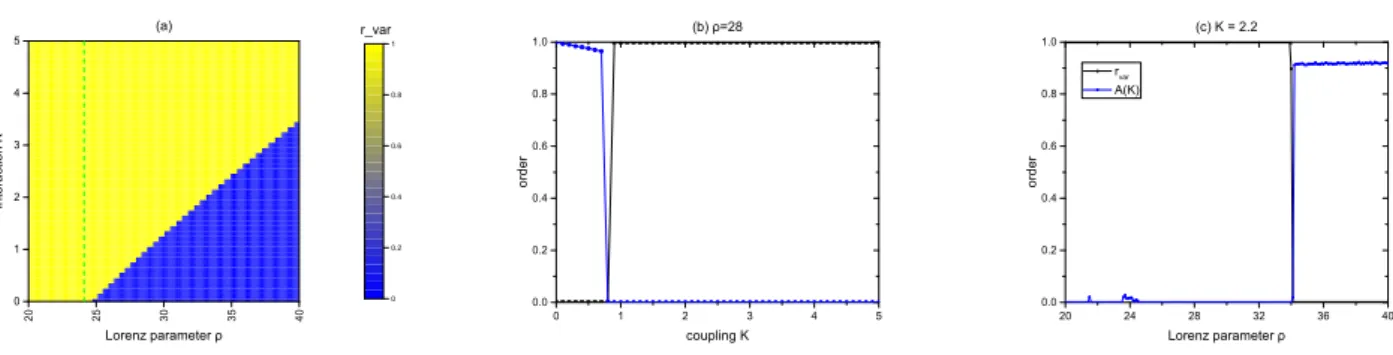Figure 9: Order parameters according to the coupling strength K and lorenz parameter ρ:

## Numerical Tests

In the previous section , a reservoir consisting of regular phase oscillators (ES) was presented. Aijsin(θj−θi), i N, (11) whereλis the coupling strength of oscillators andAij is the entry of the adjacency matrix of the network. The effect of criticality is greater when the corresponding criticality is of the first order (discontinuous) phase transition, rather than of the second order (continuous).

This section attempts to compare the performance of the above two critical reservoirs, orderly and chaotic, when both are preparing in the first-order transition phase. One possible explanation for the superiority of the chaotic reservoir is that the computational ability of critical reservoirs may depend on the collapsed dimension of reservoir attractors along the critical point. That is, the effect of criticality on computation performance can be related to how much reduction occurs in the dimension of the synchronization manifold at the phase transition.

We can check that the capacity of QC increases continuously, but the capacity of ES does not increase after about 30 nodes, which agrees with the results of the numerical tasks in Figure 11. Simulations showed that networks of phase oscillators maximize their dynamic information range processing if configured at the sync edge. Especially in the explosive synchronization, in a critical parameter regime where each frequency mode undergoes simultaneous synchronization, the computational performance is significantly improved compared to that of the regular synchronization.

However, since evaluation of the variance order parameter requires a long time to access the entire network, its measurement may be impractical, if not impossible. In sections 3 and 4 we showed that the coupled chaotic systems in the regime of quenched chaos (amplitude dead) can be used for efficient reservoir calculation. As the reservoir of the coupled phase oscillators at explosive synchronization, the chaotic reservoirs use a criticality at the first order (discontinuous) phase transition to create a ground state for computation.

Epstein, “Experimental and theoretical studies of a coupled chemical oscillator: phase dead, multistability, and in-phase and out-of-phase entrainment,” The Journal of Physical Chemistry , vol. Orriols, "Experimental observation of the amplitude dead effect in two coupled nonlinear oscillators," Physical Review Letters, vol. Xu, “Explosive death of conjugate coupled van der pol oscillators on networks,” Physical Review E, vol.

Boccaletti, "Explosive first-order transition to synchrony in networked chaotic oscillators," Physical review letters, vol. Lourenço, "Computing with chaos: A paradigm for cortical activity," Proceedings of the National Academy of Sciences, vol.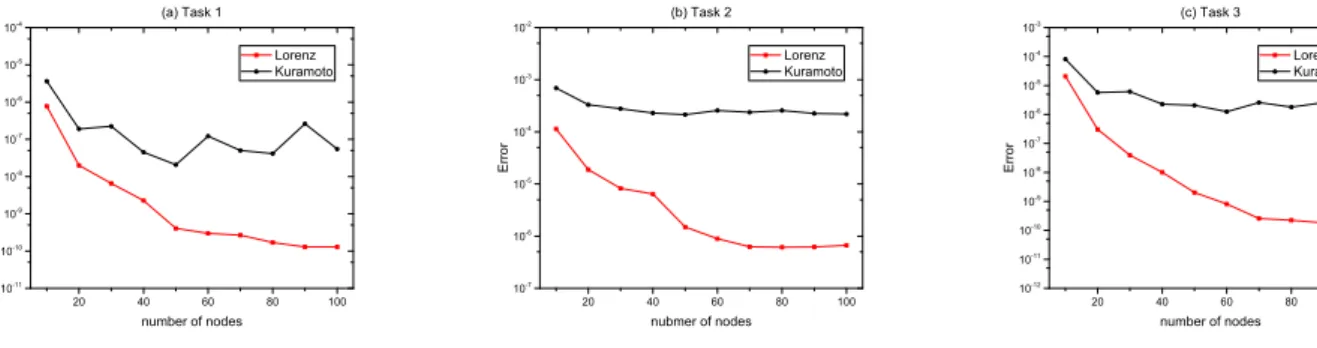Figure 11: Test errors of QC and ES with respect to number of nodes.

수치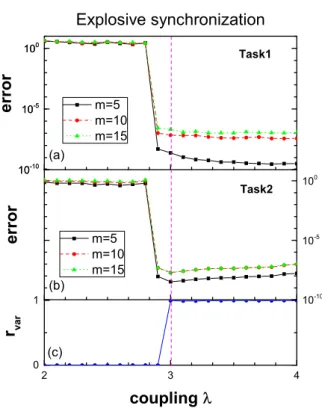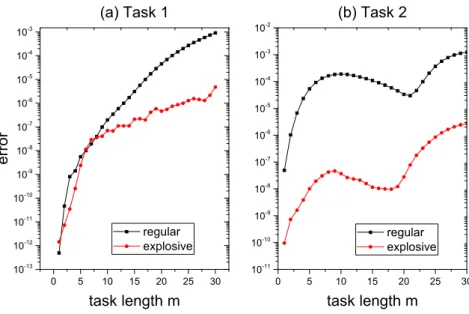+7

참조

관련 문서

Roughage proportion and intake level did not have an effect on respiration rate (RR), but ambient temperature did. Ambient temperature did not have an effect on HP, TSS and

In summary, the gas-phase reactions of MNPA show a mechanistic change as the pKa value of the nucleophile is decreased from rate-limiting formation to breakdown of T~. at a

Time-varying Fields: Faraday’s Law Introduction to Electromagnetics, 3 rd Edition, David J..

- \$23-2: The Gibbs energy of a substance has a close connection to its phase diagram -.. Comparison of the molar Gibbs energies

• If the system contains a low-temperature phase in equilibrium with a high-temperature phase at the equilibrium phase transition temperature then introduction of heat to

The previous Commissioner report (in August 2016) provided a specific update on the implementation of the “Fresh Start” Plan at the end of the first 12-month phase of

The  remainder  of  the  paper  is  organized  as  follows.  Section  2  presents  a  small  New  Keynesian  model

・ As for evaluation rank 1 (★), even if the system has not been established yet, the same level evaluation can be obtained as long as they have secured personnel, such

 Someone assigned female at birth who makes the transition to live as a man and a person assigned male at birth who makes the transition to live as a woman both share

언어 선택하기

웹사이트는 선택한 언어로 번역됩니다.

기타 언어